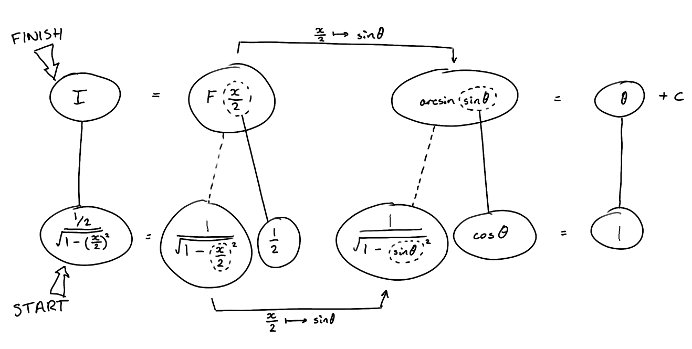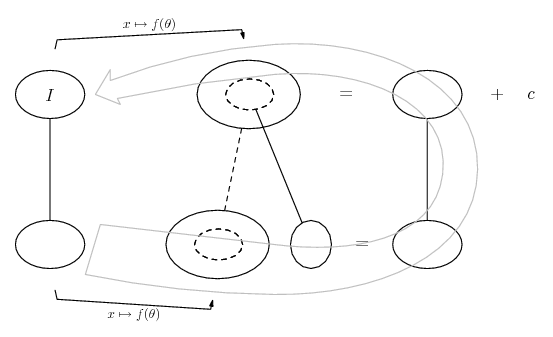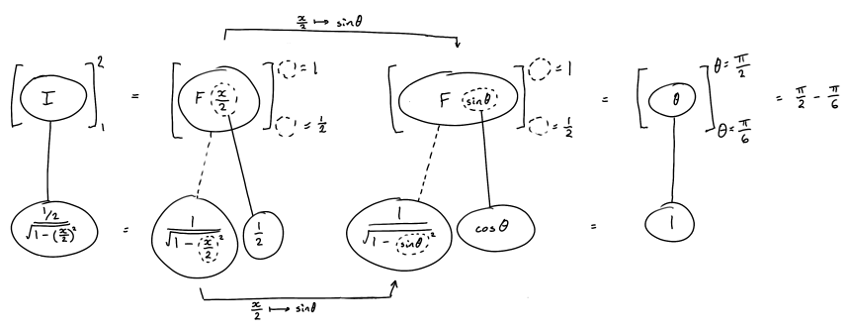1. ## integral

integral btwn 1 and 2 of 1/sqrt{4-x^2} dx

Having a tough time with this one. I tried making \sqrt{4-x^2} into the formula of a circle, but then wasnt sure what to do with the area of 1 quadrent of a circle between 1 and 2. Also tried and failed with 1/\sqrt{4(1-x^2/4)}= 1/4cos(\theta).

can anyone give some insight?

2. Just in case a picture helps with the anti-derivative...... where (key in spoiler) ...

Spoiler:... is the chain rule. Straight continuous lines differentiate downwards (integrate up) with respect to the main variable (in this case x), and the straight dashed line similarly but with respect to the dashed balloon expression (the inner function of the composite which is subject to the chain rule).

The general drift is...After the sub, cos as the inner derivative is cancelled by another cos produced courtesy of Pythag.

Edit: the definite integral...________________________________________

Don't integrate - balloontegrate!

Balloon Calculus; standard integrals, derivatives and methods

Balloon Calculus Drawing with LaTeX and Asymptote!

3.Originally Posted by frankinaroundintegral btwn 1 and 2 of 1/sqrt{4-x^2} dx

Having a tough time with this one. I tried making \sqrt{4-x^2} into the formula of a circle, but then wasnt sure what to do with the area of 1 quadrent of a circle between 1 and 2. Also tried and failed with 1/\sqrt{4(1-x^2/4)}= 1/4cos(\theta).

can anyone give some insight?
tom@ballooncalculus's methods are good, but his writing is a bit small on this one. He's saying, in a nutshell, to make the substitution x = 2*sin(theta). Once you have that his steps are pretty easy to figure out.

-Dan

4. Hello, frankinaround!

integral between 1 and 2 of 1/sqrt{4-x^2} dx

. . . . . . . . . . . . . . . ._____
First of all, .y .= .1/√4-x^2 .is not a circle.

Second, don't you recognize the arcsine integral?

#### Search Tags

integral